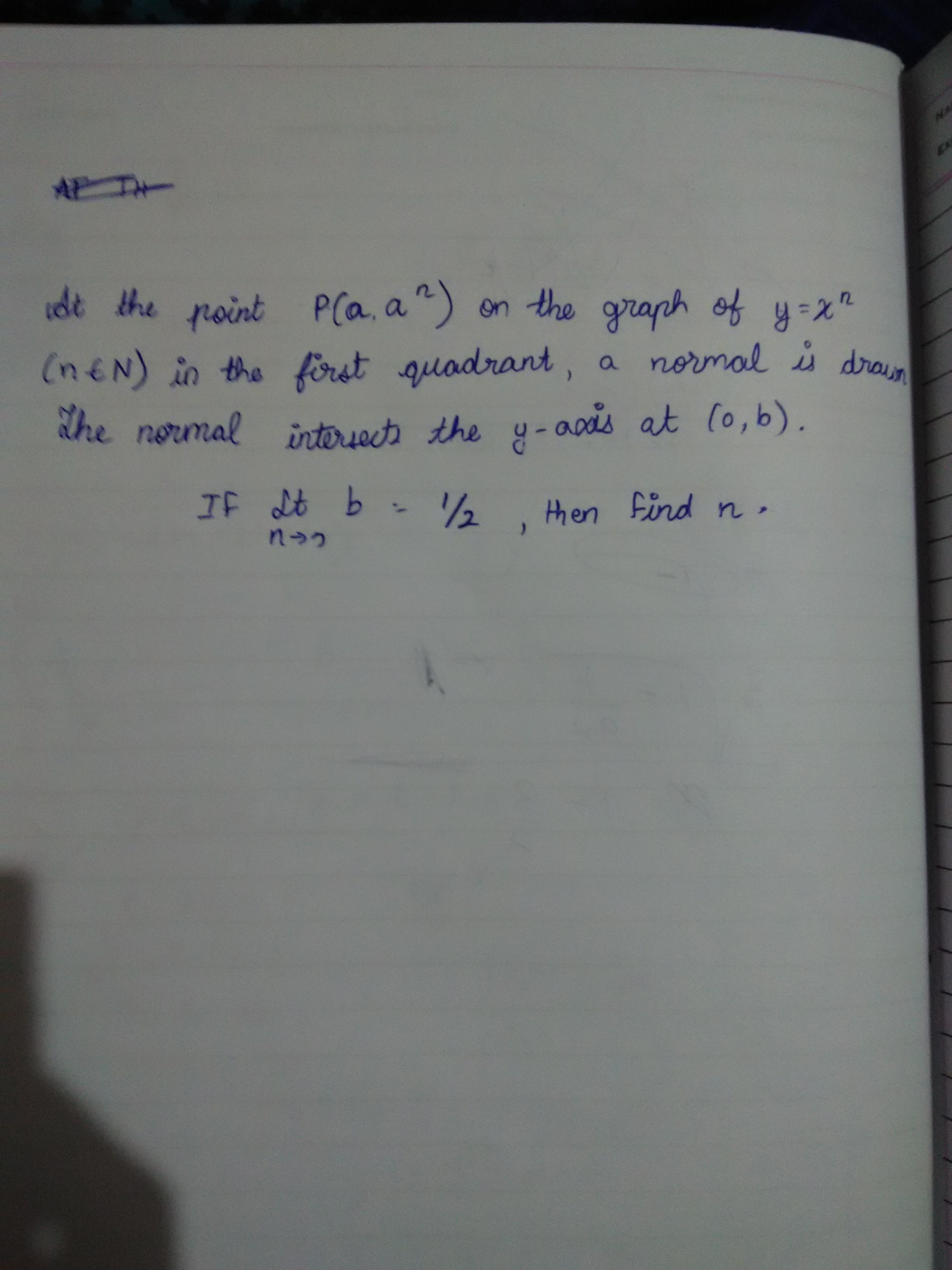# Funny Question on CalculusHello everyone. Today in class, my math teacher and me had a very long drawn debate about this question and were confused about how to solve the question. I have attached an image of the question. My doubt is whether the question is sensible asking us to find n after giving us a limit with n tending to 0. Also natural numbers don't tend to 0. Please help me out. I would appreciate it if anyone could post a solution or suggest some possible alteration to the question to obtain the correct answer. Thank you.Note by Anirudh Chandramouli
5 years, 4 months ago

This discussion board is a place to discuss our Daily Challenges and the math and science related to those challenges. Explanations are more than just a solution — they should explain the steps and thinking strategies that you used to obtain the solution. Comments should further the discussion of math and science.

When posting on Brilliant:

• Use the emojis to react to an explanation, whether you're congratulating a job well done , or just really confused .
• Ask specific questions about the challenge or the steps in somebody's explanation. Well-posed questions can add a lot to the discussion, but posting "I don't understand!" doesn't help anyone.
• Try to contribute something new to the discussion, whether it is an extension, generalization or other idea related to the challenge.

MarkdownAppears as
*italics* or _italics_ italics
**bold** or __bold__ bold
- bulleted- list
• bulleted
• list
1. numbered2. list
1. numbered
2. list
Note: you must add a full line of space before and after lists for them to show up correctly
paragraph 1paragraph 2

paragraph 1

paragraph 2

[example link](https://brilliant.org)example link
> This is a quote
This is a quote
    # I indented these lines
# 4 spaces, and now they show
# up as a code block.

print "hello world"
# I indented these lines
# 4 spaces, and now they show
# up as a code block.

print "hello world"
MathAppears as
Remember to wrap math in $$ ... $$ or $ ... $ to ensure proper formatting.
2 \times 3 $2 \times 3$
2^{34} $2^{34}$
a_{i-1} $a_{i-1}$
\frac{2}{3} $\frac{2}{3}$
\sqrt{2} $\sqrt{2}$
\sum_{i=1}^3 $\sum_{i=1}^3$
\sin \theta $\sin \theta$
\boxed{123} $\boxed{123}$

Sort by:

Since $b = a^n + \tfrac{1}{n a^{n-2}}$, perhaps the question wanted you to take the limit as $a \to 0$. This gives a limit of $\tfrac12$ when $n=2$.

- 5 years, 3 months ago

Yes even we assumed that. But can we solve the question assuming the question to be right? Thank you for posting a solution.

- 5 years, 3 months ago

Even if you could let an integer $n$ tend to 0, how would you expect to evaluate $n$ by letting it tend to $0$?

You can (with the appropriate topology) let $n$ tend to 0, but this amounts to setting $n$ equal to 0, and $b$ then does not exist for positive $a$.

The question must be incorrectly stated.

- 5 years, 3 months ago

Yes that seems very logical. I understood now. Thank you once again.

- 5 years, 3 months ago

La solucion es la siguiente

- 5 years, 3 months ago

si señor, ¿podría explicar?

- 5 years, 3 months ago

Hola tienes un email para enviarte la solucion, saludos

- 5 years, 3 months ago

si senor. anirudhvan@gmail.com gracias.

- 5 years, 3 months ago